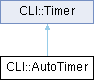CLI11 C++11 Command Line Interface Parser
CLI::AutoTimer Class Reference

This class prints out the time upon destruction. More...

#include <Timer.hpp>

Inheritance diagram for CLI::AutoTimer:Public Member Functions

AutoTimer (std::string title="Timer", time_print_t time_print=Simple)
Reimplementing the constructor is required in GCC 4.7.

~AutoTimer ()
This destructor prints the string.Public Member Functions inherited from CLI::Timer
Timer (std::string title="Timer", time_print_t time_print=Simple)
Standard constructor, can set title and print function.

std::string time_it (std::function< void()> f, double target_time=1)
Time a function by running it multiple times. Target time is the len to target.

std::string make_time_str () const
This formats the numerical value for the time string.

std::string make_time_str (double time) const
This prints out a time string from a time.

std::string to_string () const
This is the main function, it creates a string.

Timeroperator/ (std::size_t val)
Division sets the number of cycles to divide by (no graphical change)Static Public Member Functions inherited from CLI::Timer
static std::string Simple (std::string title, std::string time)
Standard print function, this one is set by default.

static std::string Big (std::string title, std::string time)
This is a fancy print function with — headers.Protected Types inherited from CLI::Timer
This is a typedef to make clocks easier to use.

using time_point = std::chrono::time_point< clock >
This typedef is for points in time.

using time_print_t = std::function< std::string(std::string, std::string)>
This is the type of a printing function, you can make your own.Protected Attributes inherited from CLI::Timer
std::string title_
This is the title of the timer.

time_print_t time_print_
This is the function that is used to format most of the timing message.

time_point start_
This is the starting point (when the timer was created)

std::size_t cycles {1}
This is the number of times cycles (print divides by this number)

Detailed Description

This class prints out the time upon destruction.

The documentation for this class was generated from the following file: